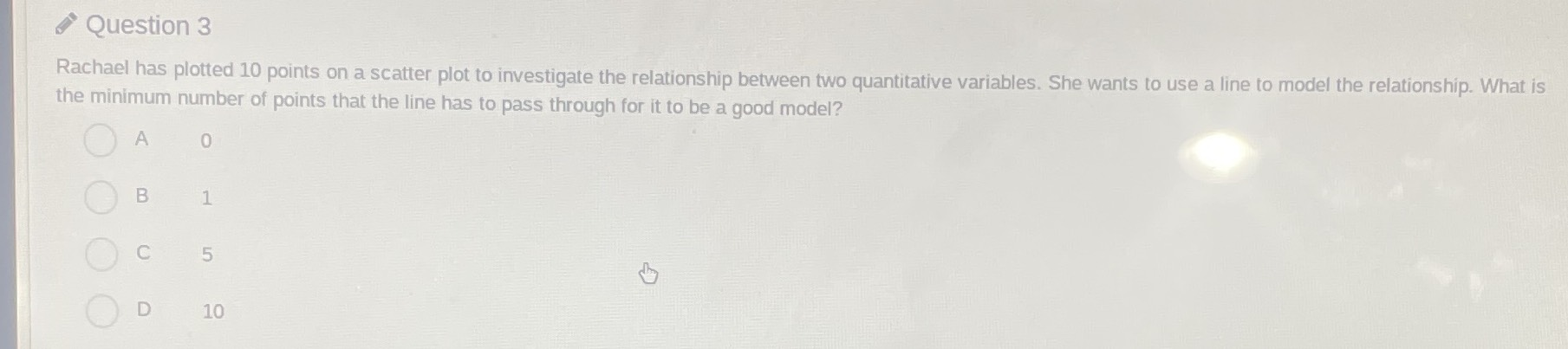### Still have math questions?

Algebra
QuestionRachael has plotted $$10$$ points on a scatter plot to investigate the relationship between two quantitative variables. She wants to use a line to model the relationship. What is the minimum number of points that the line has to pass through for it to be a good model?

$$A 0$$

$$B 1$$

$$C 5$$

$$D 10$$